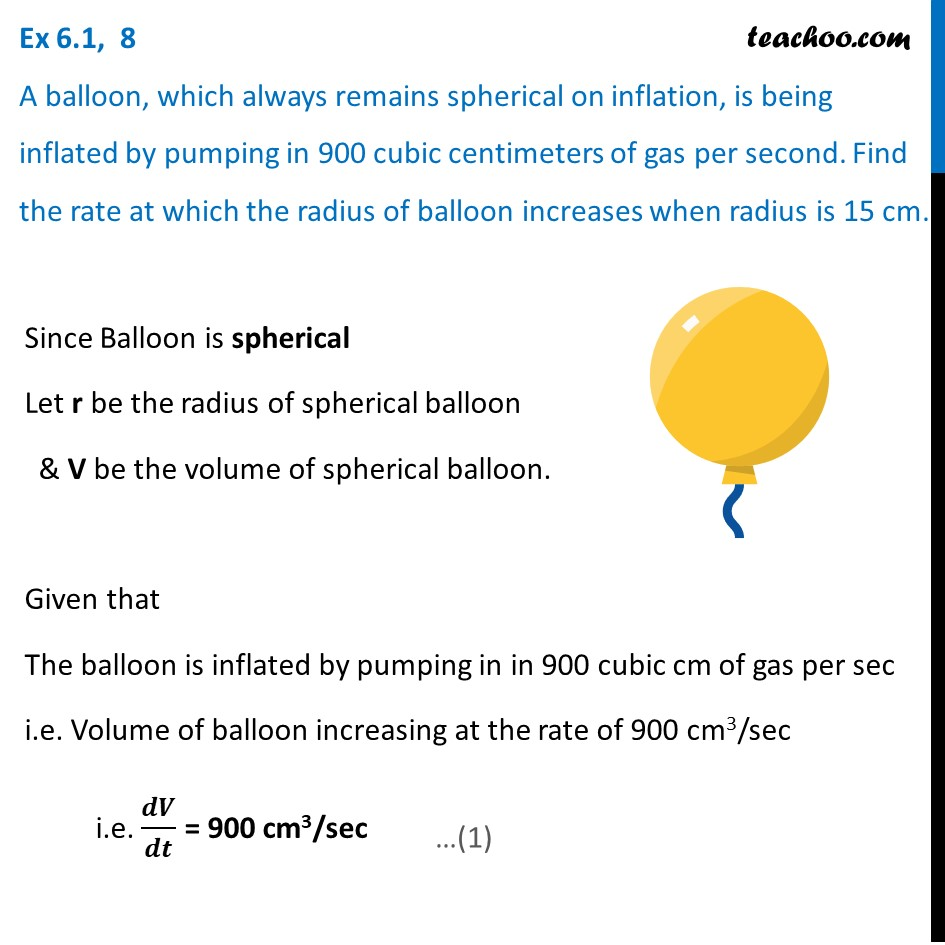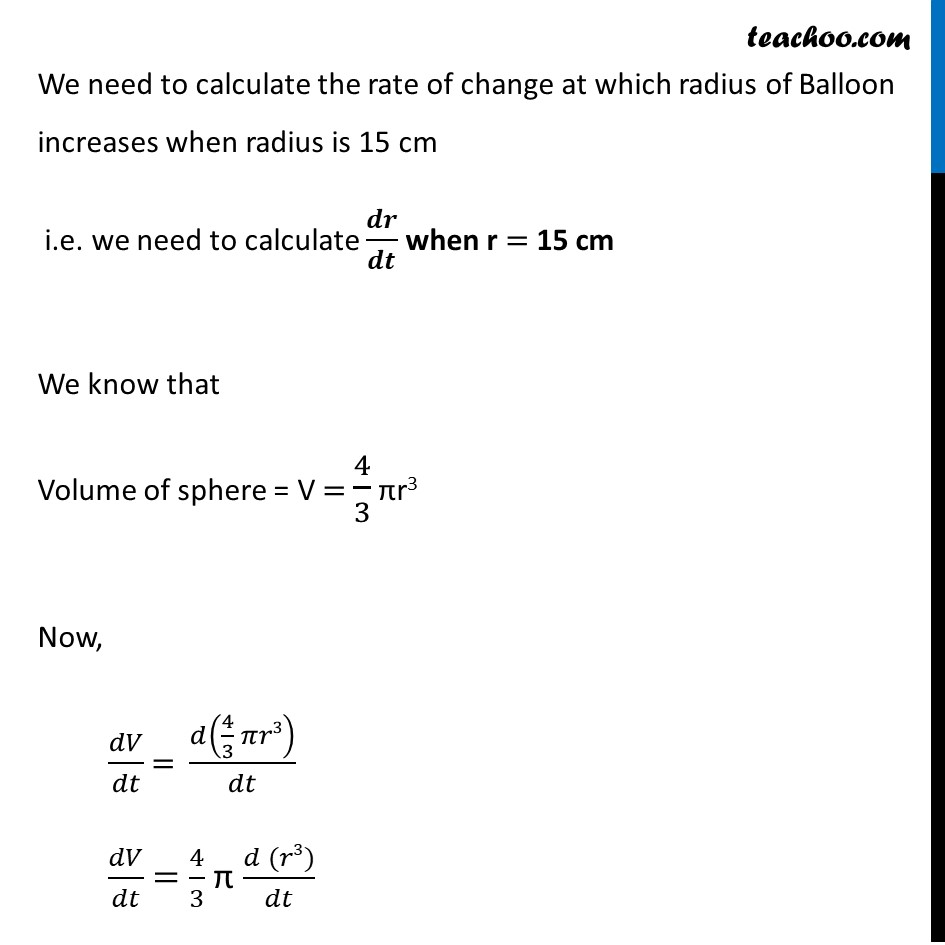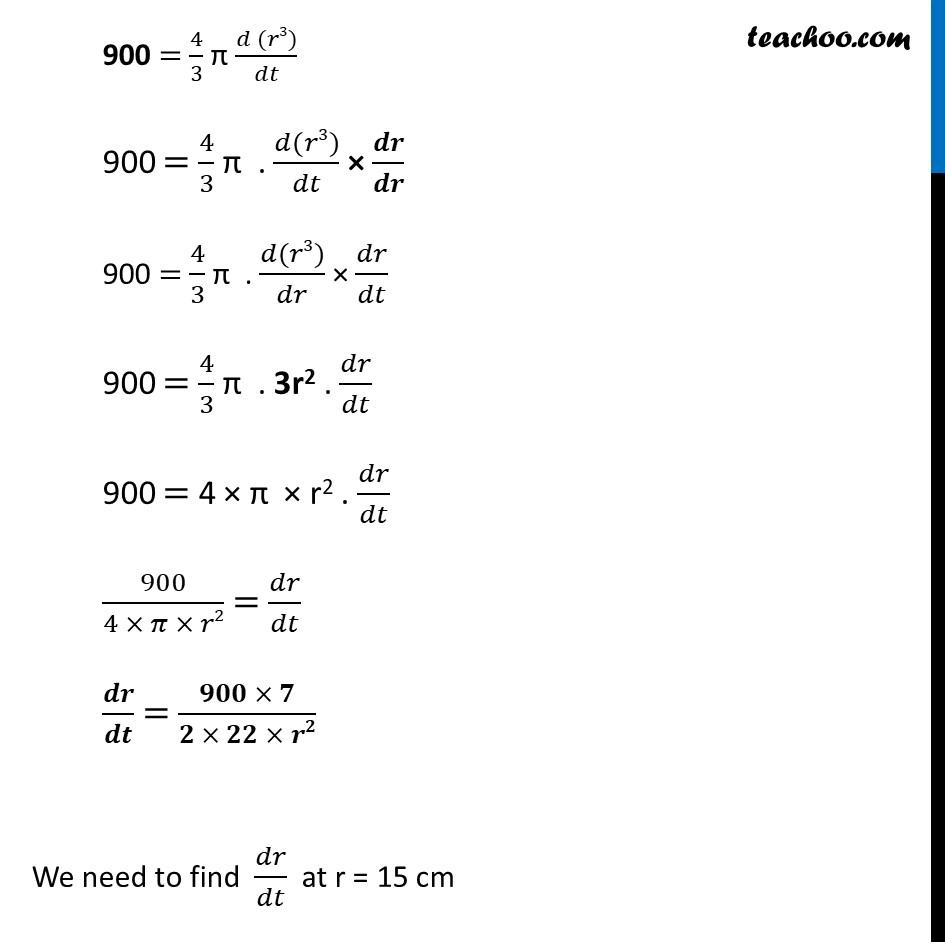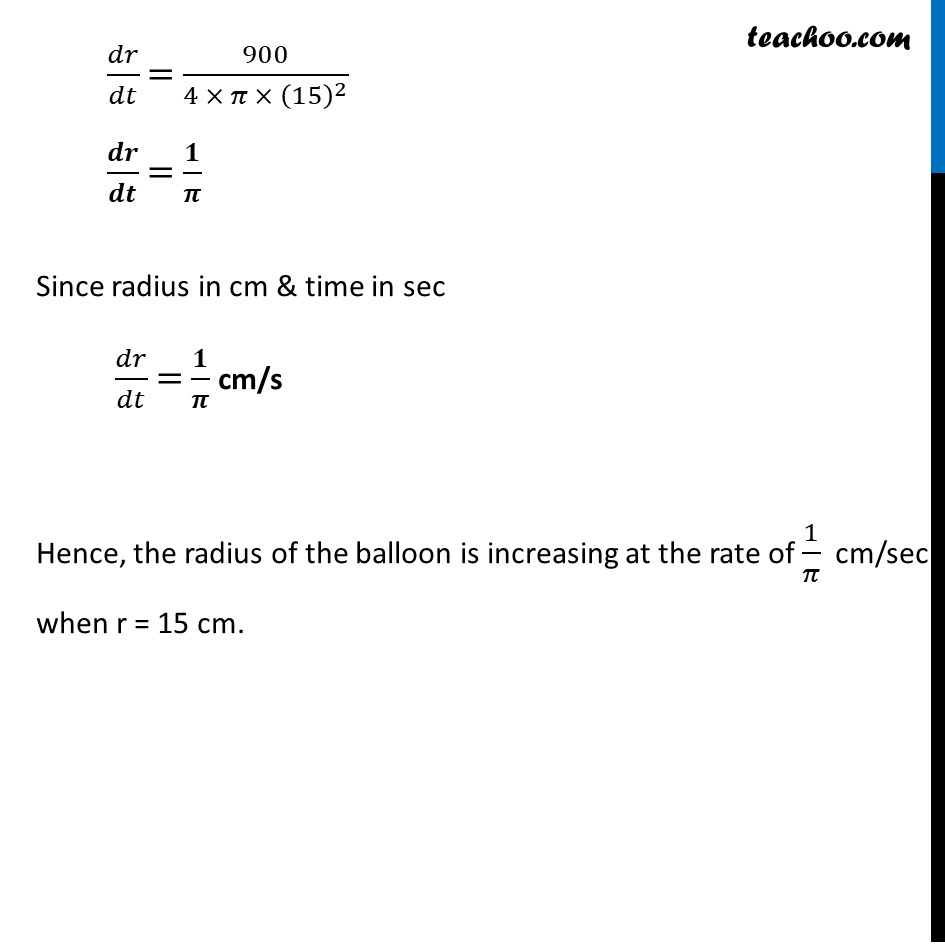Finding rate of change

Chapter 6 Class 12 Application of Derivatives (Term 1)
Concept wise### Transcript

Ex 6.1, 8 A balloon, which always remains spherical on inflation, is being inflated by pumping in 900 cubic centimeters of gas per second. Find the rate at which the radius of balloon increases when radius is 15 cm.Since Balloon is spherical Let r be the radius of spherical balloon & V be the volume of spherical balloon. Given that The balloon is inflated by pumping in in 900 cubic cm of gas per sec i.e. Volume of balloon increasing at the rate of 900 cm3/sec i.e. 𝒅𝑽/𝒅𝒕 = 900 cm3/sec We need to calculate the rate of change at which radius of Balloon increases when radius is 15 cm i.e. we need to calculate 𝒅𝒓/𝒅𝒕 when r = 15 cm We know that Volume of sphere = V = 4/3 πr3 Now, 𝑑𝑉/𝑑𝑡 = 𝑑(4/3 𝜋𝑟3)/𝑑𝑡 𝑑𝑉/𝑑𝑡 = 4/3 π (𝑑 (𝑟3))/𝑑𝑡 900 = 4/3 π (𝑑 (𝑟3))/𝑑𝑡 900 = 4/3 π . (𝑑(𝑟3))/𝑑𝑡 × 𝒅𝒓/𝒅𝒓 900 = 4/3 π . (𝑑(𝑟3))/𝑑𝑟 × 𝑑𝑟/𝑑𝑡 900 = 4/3 π . 3r2 . 𝑑𝑟/𝑑𝑡 900 = 4 × π × r2 . 𝑑𝑟/𝑑𝑡 900/(4 × 𝜋 × 𝑟2) = 𝑑𝑟/𝑑𝑡 𝒅𝒓/𝒅𝒕 = (𝟗𝟎𝟎 × 𝟕)/(𝟐 × 𝟐𝟐 × 𝒓𝟐) We need to find 𝑑𝑟/𝑑𝑡 at r = 15 cm 𝑑𝑟/𝑑𝑡 = 900/(4 × 𝜋 × (15)^2 ) 𝒅𝒓/𝒅𝒕 = 𝟏/𝝅 Since radius in cm & time in sec 𝑑𝑟/𝑑𝑡 = 𝟏/𝝅 cm/s Hence, the radius of the balloon is increasing at the rate of 1/𝜋 cm/sec when r = 15 cm.